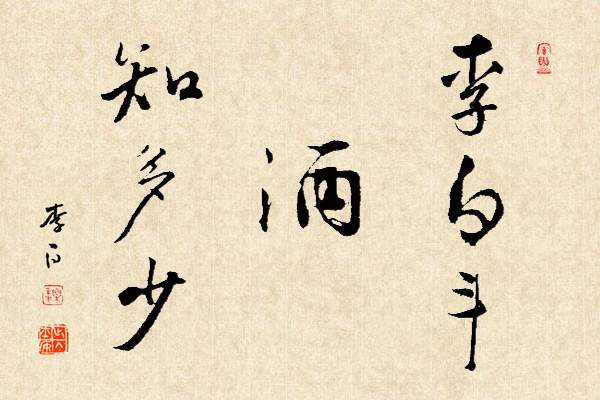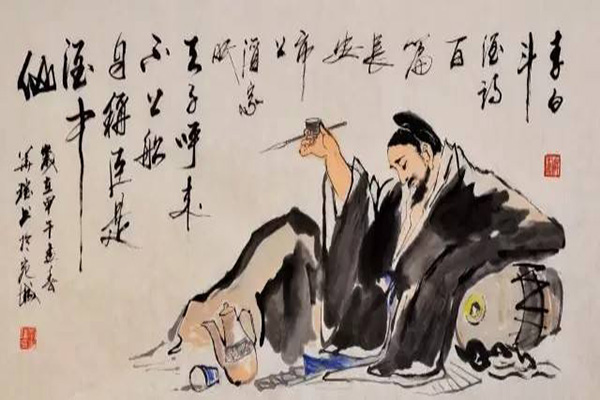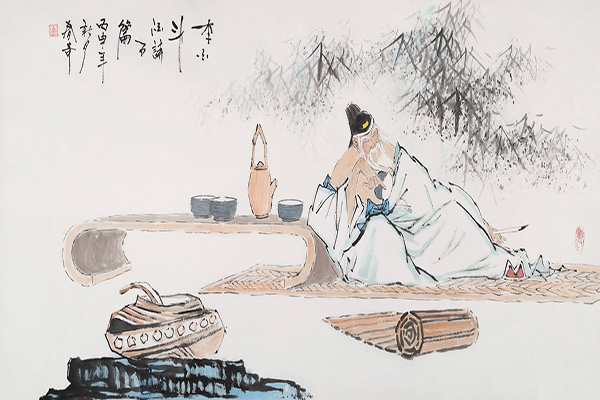(1)题中已告诉了我们先店后花的顺序，故最符合题意;

(2)店与花的先后顺序不确定，答案也应不同;(3)题目中可有多少种答案，或者说有多少种合理的答案。

1.初读文本

“遇店加一倍，见花喝一斗”，从顺序上来说，一般就是先遇店后遇花了。“三遇店和花，喝光壶中酒”，即“按照店和花”的顺序依次出现三次，运用“倒过来想”的解题策略解答如下：2.再读文本

“遇店加一倍，见花喝一斗”这句话是否就标明了遇到店和花的顺序，唐代时的语言习惯是否就是这样若是，则争论中(1)的答案就是正确的。但我们从数学的角度，不妨也放开思维来思考，“这句话”是否可作为题目里的条件，如果把此题当作一个游戏，这即是一个“规则”，规则是并列的关系，则“三遇店和花，喝光壶中酒”就具有无序性，是店店店花花花，还是店花店花店花，还是花店花店花店……这都有可能性，也就是题目是开放的，那就有多种答案的可能。3.三读文本

(1)BBBA①(0+1)÷2=0.5;②0.5÷2÷2=0.125;③0.125+1+1=2.125(斗)

(2)ABABBA①(0+1)÷2=0.5;②0.5÷2+1=1.25;③1.25÷2+1=1.625(斗)

(3)ABBABA①(0+1)÷2=0.5;②(0.5+1)÷2=0.75;③0.75÷2+1=1.375(斗)

(4)ABBB①0+1+1=2;②2÷2÷2=0.5;③0.5÷2+1=1.25(斗)

(5)BBBA①(0+1)÷2=0.5;②0.5÷2+1=1.25;③(1.25+1)÷2=1.125(斗)

(6)BBBA①(0+1)÷2=0.5;②0.5+1+1=2.5;③2.5÷2÷2=0.625(斗)

(7)BBBA①0+1+1=2;②(2+1)÷2=1.5;③1.5÷2÷2=0.375(斗)

(8)BABB①0+1+1=2;②2÷2÷2=0.5;③(0.5+1)÷2=0.75(斗)

(9)BABABA①(0+1)÷2=0.5;②(0.5+1)÷2=0.75;③(0.75+1)÷2=0.875(斗)

(10)BBAB①0+1+1=2;②2÷2+1=2;③2÷2÷2=0.5(斗)

4.四读文本

“李白街上走，提壶去打酒。”从“提壶去打酒”这句话可知，壶里酒是不多的，但也不可能没有酒，故“1.125、1.25、1.375、1.625、2.125”这五种答案是不符合题意的，即打酒的过程中首次不能遇到花。试想，诗仙李白怎么能提着较多酒的酒壶再去打酒呢!“斗”是“酒具”还是“容积单位”为什么说李白不应提着较多酒的壶去打酒，或不说是提大半壶酒去打酒呢通过文本细读，我们又把解读的重点放到了“斗”这个字上，这里的“斗”是什么意思呢教科书上说，斗是酒具，也是一种容积单位，但没有指明文中的斗是指什么。我们查阅资料发现有“壶、觫、尊、樽、觞、钟、斗、酌、爵、白、角、觯、杯杓、觚、杯”等酒具，说明“斗”可能是酒具或是盛酒的容器。